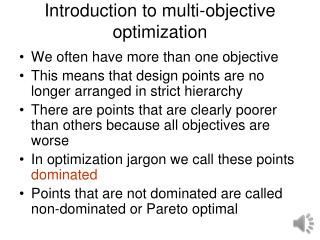Download PresentationIntroduction to multi-objective optimization

# Introduction to multi-objective optimization - PowerPoint PPT PresentationDownload Presentation## Introduction to multi-objective optimization

- - - - - - - - - - - - - - - - - - - - - - - - - - - E N D - - - - - - - - - - - - - - - - - - - - - - - - - - -
##### Presentation Transcript

1. Introduction to multi-objective optimization • We often have more than one objective • This means that design points are no longer arranged in strict hierarchy • There are points that are clearly poorer than others because all objectives are worse • In optimization jargon we call these points dominated • Points that are not dominated are called non-dominated or Pareto optimal

2. Vilfredo Pareto, 1848-1923 The Italian economist Vilfredo Pareto was one of the leaders of the Lausanne School and an illustrious member of the "second generation" of the Neoclassical revolution.  Although only mildly influential during his lifetime, his "tastes-and-obstacles" approach to general equilibrium theory were resurrected during the great "Paretian Revival" of the 1930s and have guided much of economics since.

3. Definition of Pareto optimality • For a problem with m objective functions, a design variable vector x* is Pareto optimal if and only if there is no vector xin the feasible space with the characteristics

4. Example Minimize time so that you make at least \$100 and maximize fun. Will need between 33.3 to 100 items. Time can vary from 66.7 minutes to 300. Fun can vary between 33.3 and 300.

5. Example Item 2 is dominated. Items 1,3,4 are Pareto optimal

6. Multi-objective Formulation

7. Solution methods • Methods that try to avoid generating the Pareto front • Generate “utopia point” • Define optimum based on some measure of distance from utopia point • Generating entire Pareto front • Weighted sum of objectives with variable coefficients • Optimize one objective for a range of constraints on the others • Niching methods with population based algorithms

8. The utopia point is (66.7,300). Finding the nearest point may be a reasonable compromise. The entire front tells us, that for this problem, the front is almost a straight line, so there is no clear appealing compromise.

9. Series of constraints

10. Matlab segment x0 = [10 10 10 10]; for fun_idx = 30:5:300 A = [-1 -2 -1 -3; -3 -2 -2 -1]; b = [-100;-fun_idx]; lb = zeros(4,1); options = optimset('Display','off'); [x,fval,exitflag,output,lambda] = fmincon('myfun',x0,A,b,[],[],lb,[],[],options); pareto_sol(fun_idx,:) = x; pareto_fun(fun_idx,1) = fval; pareto_fun(fun_idx,2) = 3*x(1) + 2*x(2) + 2*x(3) + x(4); End function f = myfun(x) f = 3*x(1) + 4*x(2) + 2*x(3) + 2*x(4);